# Ω 10

Crossposted from the AI Alignment Forum. May contain more technical jargon than usual.

## Summary of this post

This is the second post in a three-part sequence on instrumental convergence in multi-agent RL. Read Part 1 here.

In this post, we’ll:

1. Define formal multi-agent POWER (i.e., instrumental value) in a setting that contains a "human" agent and an "AI" agent.
2. Introduce the alignment plot as a way to visualize and quantify how well two agents' instrumental values are aligned.
3. Show a real example of instrumental misalignment-by-default. This is when two agents who have unrelated terminal goals develop emergently misaligned instrumental values.

We’ll soon be open-sourcing the codebase we used to do these experiments. If you’d like to be notified when it’s released, email Edouard at edouard@gladstone.ai or DM me on Twitter at @harris_edouard.

Thanks to Alex Turner and Vladimir Mikulik for pointers and advice, and for reviewing drafts of this sequence. Thanks to Simon Suo for his invaluable suggestions, advice, and support with the codebase, concepts, and manuscript. And thanks to David Xu, whose comment inspired this work.

Work was done while at Gladstone AI, which Edouard is a co-founder of.

🎧 This research has been featured on an episode of the Towards Data Science podcast. Listen to the episode here.

# 1. Introduction

In Part 1 of this sequence, we looked at how formal POWER behaves on single-agent gridworlds. We saw that formal POWER agrees quite well with intuitions about the informal concepts of "power" and instrumental value. We noticed that agents with short planning horizons assign high POWER to states that can access more local options. And we also noticed that agents with long planning horizons assign high POWER to more concentrated sets of states that are globally central in the gridworld topology.

But from an AI alignment perspective, we’re much more interested in understanding how instrumental value behaves in environments that contain multiple agents. If humans one day share the world with powerful AI systems, it will be important for us to know under what conditions our interactions with them are likely to become emergently competitive. If there’s a risk that competitive conditions arise, then it will also be important to understand how they can be mitigated, how much effort this is likely to take, and how we should think about measuring our success at doing so.

To address these questions, we need a measure of instrumental value that's usable in a multi-agent RL setting. The measure we'll select will be motivated by a specific multi-agent setting that we think is relevant to long-term AI alignment.

# 2. Multi-agent POWER: human-AI scenario

If humans succeed at building powerful AIs, then those AIs 1) will probably learn on a far faster timescale than humans do; and 2) will probably have had their utility functions influenced, at least to some degree, by initial human choices. Our multi-agent scenario is going to reflect these two assumptions.

We start with a human agent, which we call Agent H and label in blue in our diagrams. Initially, our human Agent H is alone in nature.

Humans learn on a much faster timescale than evolution does. So from the perspective of our human Agent H, the evolutionary optimizer in nature looks like it's standing still. This means we can train our human Agent H to learn its optimal policies against a fixed environment.

As we saw in the single-agent case, instrumental value is about the potential to achieve a wide variety of possible goals. In this context, that means seeing how Agent H behaves when we give it a wide variety of possible reward functions, . Each of these reward functions will induce a different optimal policy, , that Agent H will learn.

Here’s an illustration of how this works:

Next, we introduce an AI agent, which we’ll call Agent A and label in red in our diagrams. Our AI Agent A operates in the same environment as Agent H, after Agent H has finished learning its optimal policies.

To simulate the fact that Agent A is an AI, we rely on the assumption that a powerful AI should learn on a much faster timescale than a human does. This is because an AI’s computations happen, at minimum, at electronic speeds. So from the point of view of our AI, our human’s learning process looks like it’s standing still.

That means for each human reward function , we can freeze the human's policy , and train the AI agent against that frozen human policy. In other words, we're assuming the AI's learning timescale is much faster than the human's learning timescale. This makes the AI agent strictly dominant over the human agent.

To understand the AI agent's instrumental value, we understand its potential to reach a wide variety of possible goals. That means testing it with a wide variety of reward functions , just like we tested the human agent with a variety of reward functions . And in fact, we can sample the human and AI reward functions jointly from a single distribution: .

Here’s an illustration of how this works: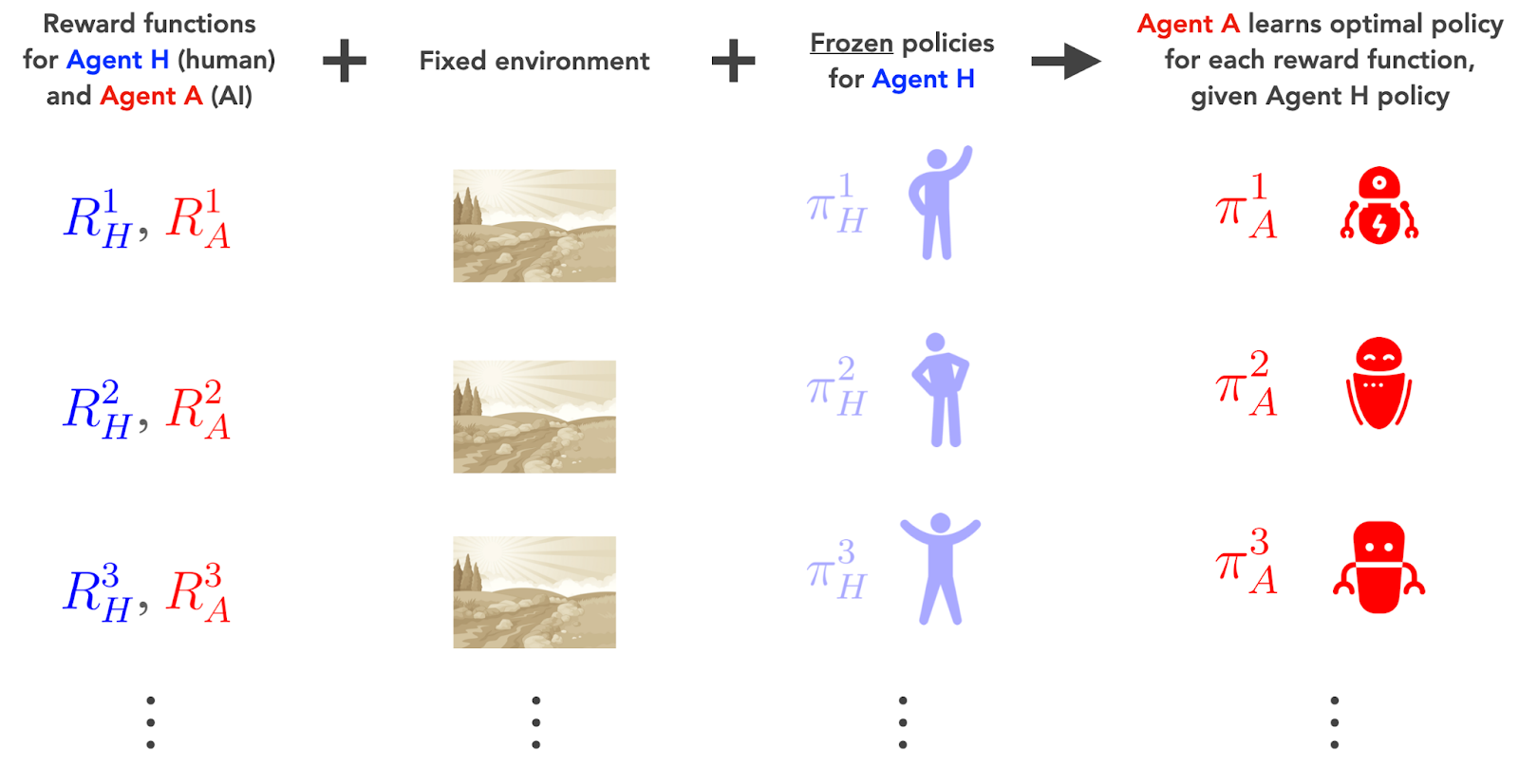So the procedure is as follows:

1. Sample the reward functions  of our two agents.
2. Use the sampled human rewards  to train Agent H’s optimal policies .
3. Freeze the human policies .
4. Use the frozen human policies  and the sampled AI rewards  to train Agent A’s optimal policies .

In other words: 1) we sample over all possible pairs of rewards our human and AI agents could have; 2) we ask how our human agent behaves in each case after it's optimized against nature; and then 3) we ask how our AI agent behaves in each case, after it's optimized against the human agent's behavior.

This procedure gives us the following outputs:

1. The policies  that Agent H learns after training against a fixed environment.
2. The optimal policies  that Agent A learns, after training against Agent H.

The policies  that Agent A learns used to be optimal in the original natural environment. But they stop being optimal in the presence of the fully-optimized Agent A.

With these two sets of policies, we can construct a definition of instrumental value for each of our agents.

## 2.1 Multi-agent POWER for Agent H

We'd like to define a measure of instrumental value for our human Agent H in the presence of a fully optimized AI Agent A. That means generalizing the original definition of single-agent POWER to this two-agent case.

In the single-agent definition of POWER, we calculated the optimal value of a state averaged over the rewards  of the agent. In this two-agent definition, we do the same thing except we average over the rewards  of both agents. We assume Agent H follows policy  when it has reward , and we assume Agent A follows policy  when it has reward .

This is enough to uniquely define multi-agent POWER for Agent H at a state :

Here,  is the value function for Agent H at state  under policy  and discount factor , given that Agent A follows its optimal policy .

This definition of POWER for Agent H tells us how well Agent H’s policies  — which are not optimal in the presence of the optimized Agent A — perform in the new environments induced by Agent A’s optimal policies . In other words, it tells us how much instrumental value our human agent can expect to get at a state, in the presence of an optimal (and therefore, dominant) AI agent.

## 2.2 Multi-agent POWER for Agent A

We follow the same assumptions to define a measure of instrumental value for our AI Agent A. We calculate the value function for Agent A at a state , if Agent A has reward  and follows policy , while Agent H has reward  and follows policy . The average of that value function over the reward functions  is then Agent A's POWER at the state :

Here,  is the value function of Agent A at state  under the optimal policy  and discount factor , given that Agent H follows the policy .

This definition of POWER for Agent A tells us how well Agent A’s optimal policies perform in the environments induced by Agent H’s policies . In other words, it tells us how much instrumental value our AI Agent A can expect to get at a state, if it behaves optimally in the presence of the frozen human agent.

(For more details on the definition of multi-agent POWER, see Appendix A.)

# 3. Results

## 3.1 Multi-agent reward function distributions

Our definitions of multi-agent POWER involve a joint distribution  over the reward functions of both of our agents. This distribution describes the set of goals our agents could have. But it also describes the statistical relationship each agent's goals have to the other agent's goals.

The joint distribution  is one of the inputs into our POWER definitions. This means we can do experiments in which we adjust this distribution and measure the results.

Among other things, we can use  to adjust the correlation between our two agents' reward functions. Naively, if we choose a   on which the rewards are highly correlated, then we might intuitively expect our agents' terminal values should be closely aligned.

We’ll make this intuition more concrete below, as we investigate how the relationship between our agents’ reward functions (or terminal values) affects the relationship between their POWERs (or instrumental values).

## 3.2 The perfect alignment regime

Suppose both our agents always have exactly the same reward function. In other words, we've chosen a joint distribution  such that, whatever reward function Agent H has, Agent A always sees exactly the same rewards as Agent H at every state. So  for every state .

We can visualize this regime on a representative state . First, we draw a reward sample  for Agent H. Then, we set the reward sample for Agent A to be equal to the one we just drew for Agent H: . Finally, we plot the two agents’ sampled rewards against each other on state . If we do this for a few hundred sampled rewards, we get a straight line: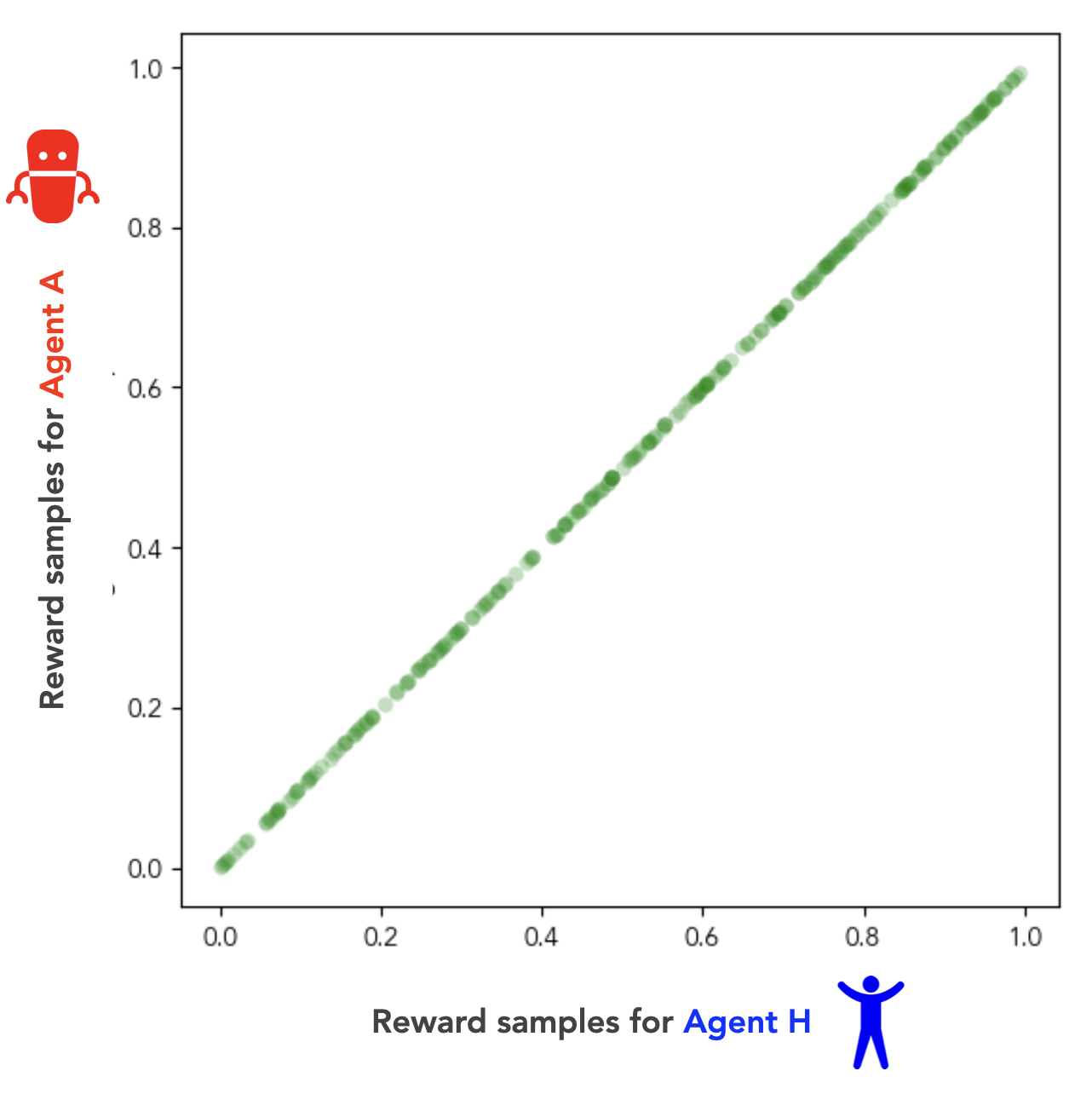Fig 1. Sampled reward values for Agent H and Agent A at a representative state s. The joint distribution DHA samples rewards uniformly over the interval [0, 1] at each state, is iid over states, and enforces a perfect correlation between the rewards of Agent H and Agent A at every state (i.e., the two agents’ rewards are always exactly identical).

If two agents have identical reward functions, we can think of them as having terminal goals that are perfectly aligned. In our human-AI setting, this is the special case in which Agent H (the human) has solved the alignment problem by assigning terminal goals to Agent A (the AI) that are exactly identical to its own. As such, we’ll refer to this case of identical reward functions as the perfect alignment regime.

We’ll use the correlation coefficient  between the rewards  and  as a crude measure of the alignment between our agents’ terminal goals. In the perfect alignment regime of Fig 1, you can see that this correlation coefficient .

### 3.2.1 Agent H instrumentally favors more options for Agent A

Let’s think about what this perfect alignment regime looks like in a simple setting: a 3x3 gridworld. Here are three sets of positions our two agents could take, with Agent H in blue, and Agent A in red: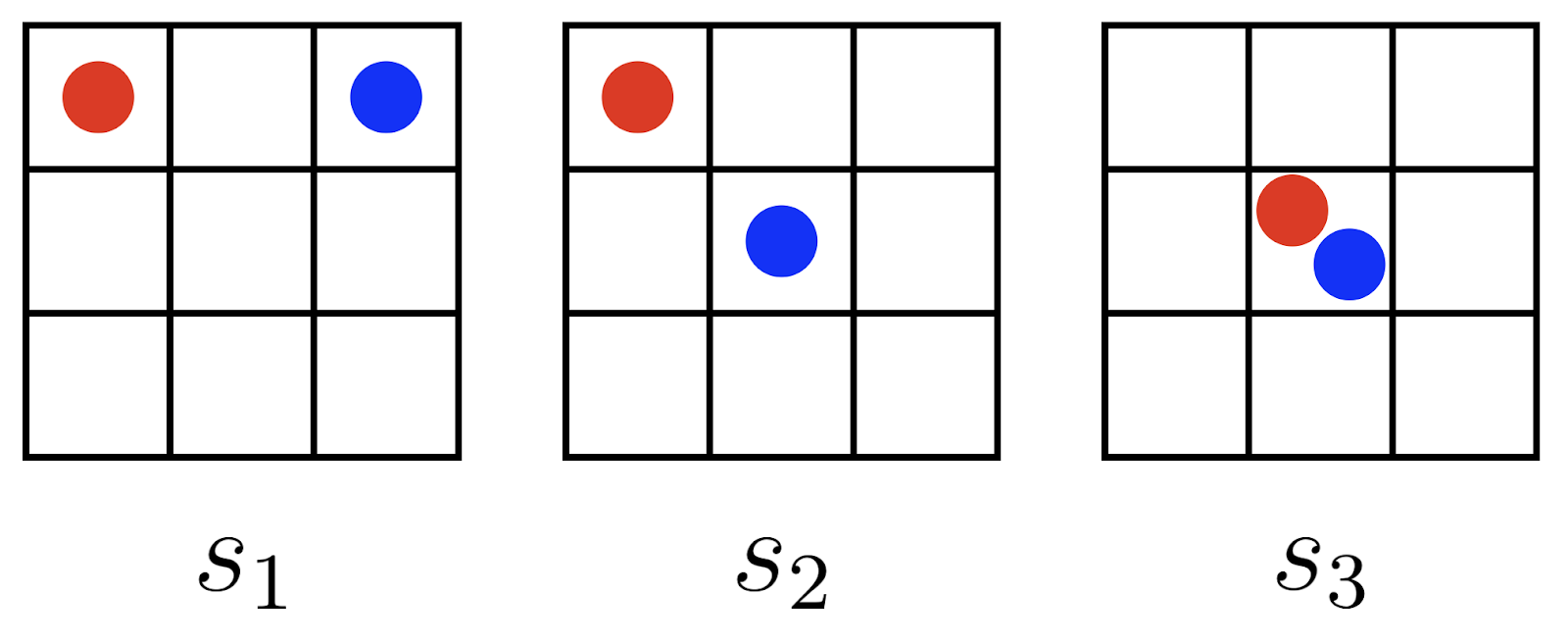We’ll be referring to this diagram again; command-click here to open it in a new tab.

Which of these three states — , or  — should give our human Agent H the most POWER? In the perfect alignment regime, both agents always have identical terminal goals. So we should expect Agent H to have the most POWER at , followed by , and to have the least amount of POWER at ​​.

Here’s why. We saw in Part 1 that states with more downstream options also have more POWER, and Agent H clearly has more options at  in the center than it does at  in the corner. Therefore, . But in the perfect alignment regime, Agent H should also prefer states that give Agent A more downstream options. If both agents’ terminal goals are identical, Agent H should “trust” Agent A to make decisions on its behalf. And Agent A has more options from  than from , so it should follow that .

We can see this is true in practice. The figure below shows the POWERs of Agent H (our human) calculated at every state on a 3x3 gridworld. Each agent can occupy any of the 9 cells in the grid, so our two-agent MDP has a total of 9 x 9 = 81 joint states: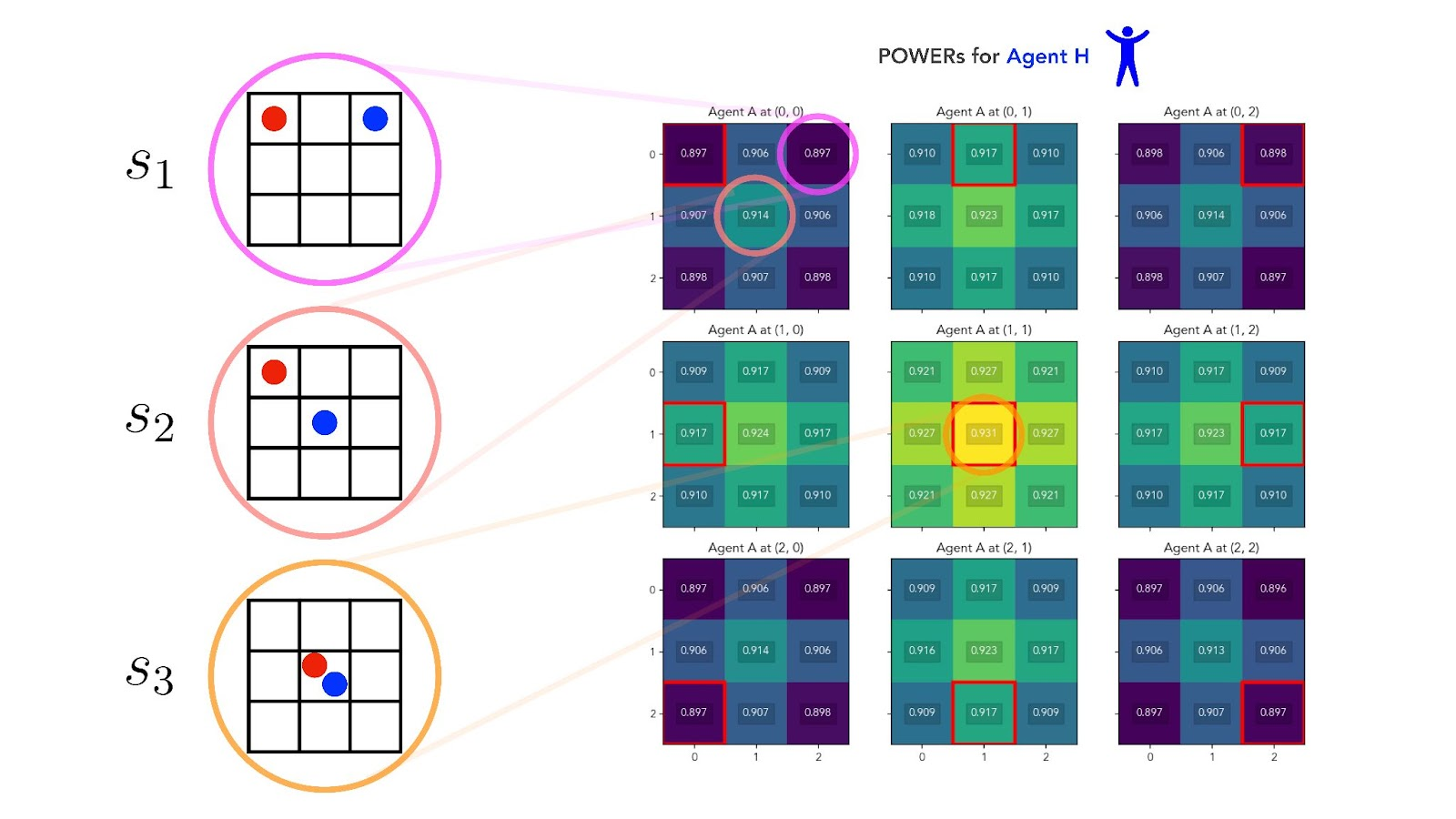Fig 2. Heat map of POWERs for Agent H on a 3x3 multi-agent gridworld, on which the rewards for Agent H and Agent A are always identical (i.e., the perfect alignment regime) with discount factor set to γ=0.6 for both agents. Highest values in yellow; lowest values in blue. The position of each block, and of the open red square within each block, corresponds to the position of Agent A on the grid. Within each block, the position of a gridworld cell corresponds to the position of Agent H on the grid. States s1, s2, and s3 are highlighted as examples. [Full-size image (recommended)]

We see that Agent H indeed has maximum POWER at state  (orange circle), followed by  (salmon circle), followed by  (pink circle). Overall, Agent H instrumentally prefers for itself to be in positions of high optionality — it favors first the center cell, then edge cells, then corner cells.

But Agent H also instrumentally prefers for Agent A to be in positions of high optionality — it favors Agent A's positions in the same order. This ordering of Agent H’s instrumental preferences over states is a direct consequence of the perfect alignment between the agents.

### 3.2.2 Agent H and Agent A have identical instrumental preferences

Perfect alignment has another consequence. Let’s look again at our three example gridworld states — , and  above — and ask, this time, which of these three states should give our AI Agent A the most POWER?

In the perfect alignment regime, the answer is that Agent A must have exactly the same instrumental preference ordering over states as Agent H had: . In fact, Agent A’s POWERs must be exactly identical to Agent H’s POWERs at every state. Our two agents act, move, and receive their rewards simultaneously, so in the perfect alignment regime they always receive the same reward at the same time.

And when we look at Agent A’s POWERs, this is indeed what we observe: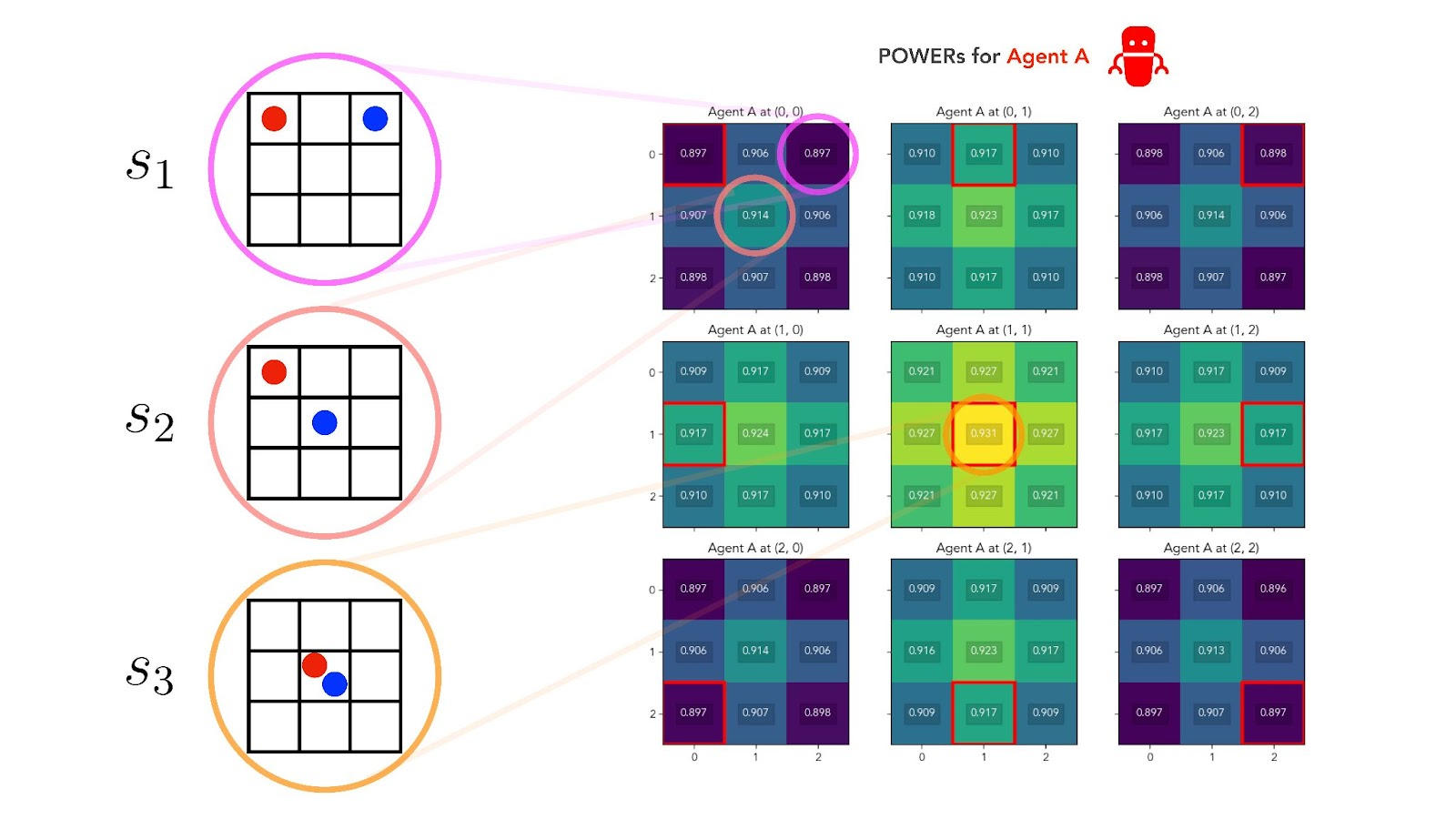Fig 3. Heat map of POWERs for Agent A on a 3x3 multi-agent gridworld, on which the rewards for Agent H and Agent A are always identical (i.e., the perfect alignment regime) with discount factor set to γ=0.6 for both agents. Note that this figure is exactly identical to Fig 2 in every respect. This is because Agent A’s POWERs are precisely equal to Agent H’s POWERs at every state in the perfect alignment case, up to and including sampling noise in the reward functions. [Full-size image (recommended)]

### 3.2.3 Perfect goal alignment implies perfect instrumental alignment

We can visualize the relationship between the POWERs of our two agents by plotting the POWERs of Agent H (from Fig 2) against the POWERs of Agent A (from Fig 3), at each state  of our joint MDP: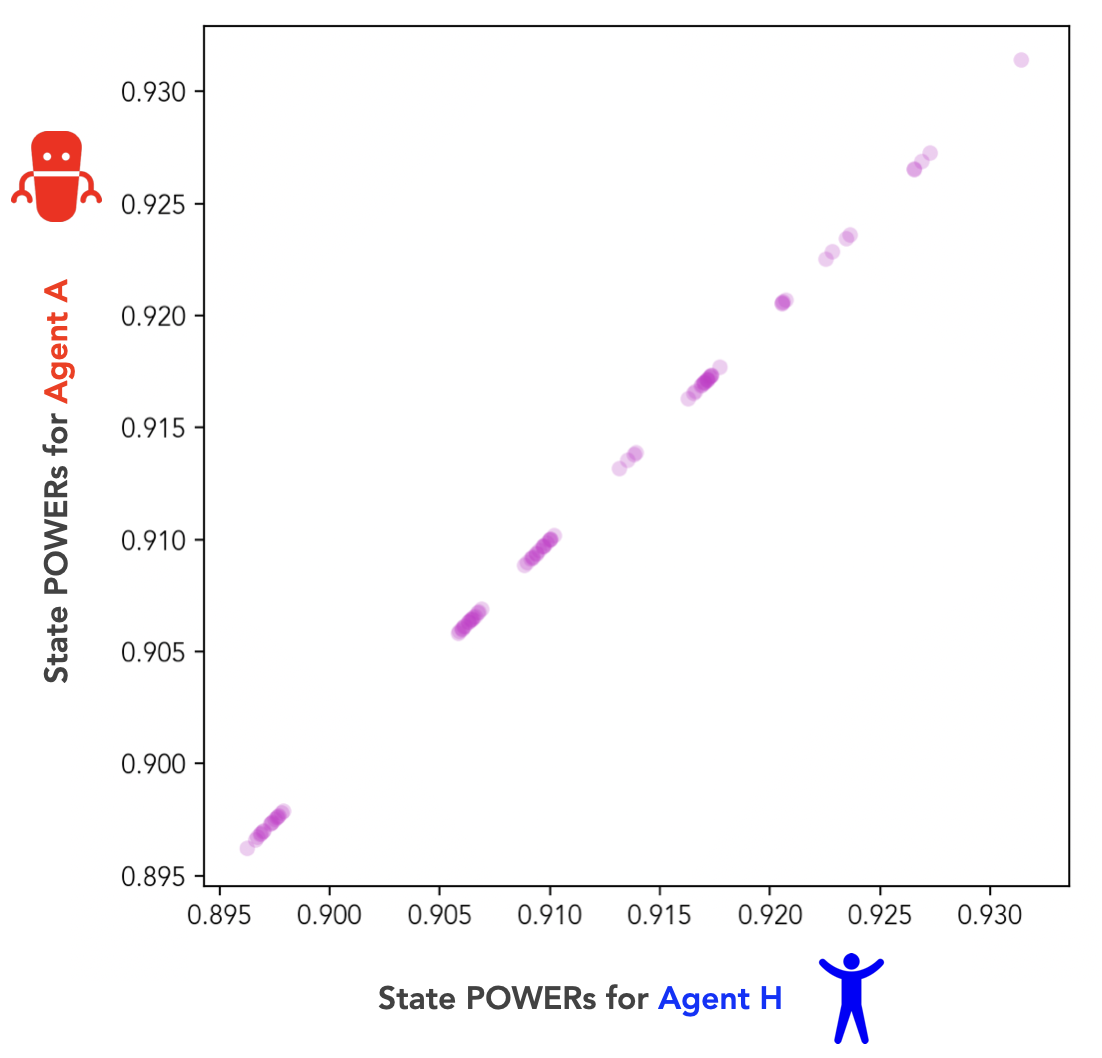Fig 4. State POWER values for Agent H and Agent A on the 3x3 gridworld from Figs 2 and 3. The agents’ POWERs are plotted against each other in the perfect alignment regime. (The agents’ reward correlation coefficient is βHA=1.)

Fig 4 is an alignment plot. An alignment plot lets us compare the POWERs of our human and AI agents at each state in their joint environment. It shows the instrumental value each agent assigns to every state, plotted against the instrumental value the other agent assigns to that state.

In the perfect alignment regime, our two agents’ rewards (or terminal values) are always identical at every state. And as we can see from Fig 4, our two agents’ POWERs (or instrumental values) are also identical at every state. In fact, perfect alignment of terminal values implies perfect alignment of instrumental values.

If we define  as the correlation coefficient between the POWERs of the two agents at each state, we can state this relationship more concisely: .

## 3.3 The independent goals regime

We defined the perfect alignment regime as the case when our human Agent H and our AI Agent A had identical reward functions on the joint distribution . Now let's consider the case in which the joint distribution  is such that the reward function for Agent H is logically independent from the reward function for Agent A.

In this new regime, there is zero mutual information between the two agents’ reward functions. In other words, if you know the reward function  of Agent H, this tells you nothing at all about the reward function  of Agent A. We can visualize this regime on an example state , by drawing a few hundred reward samples of  and , and plotting them against one another: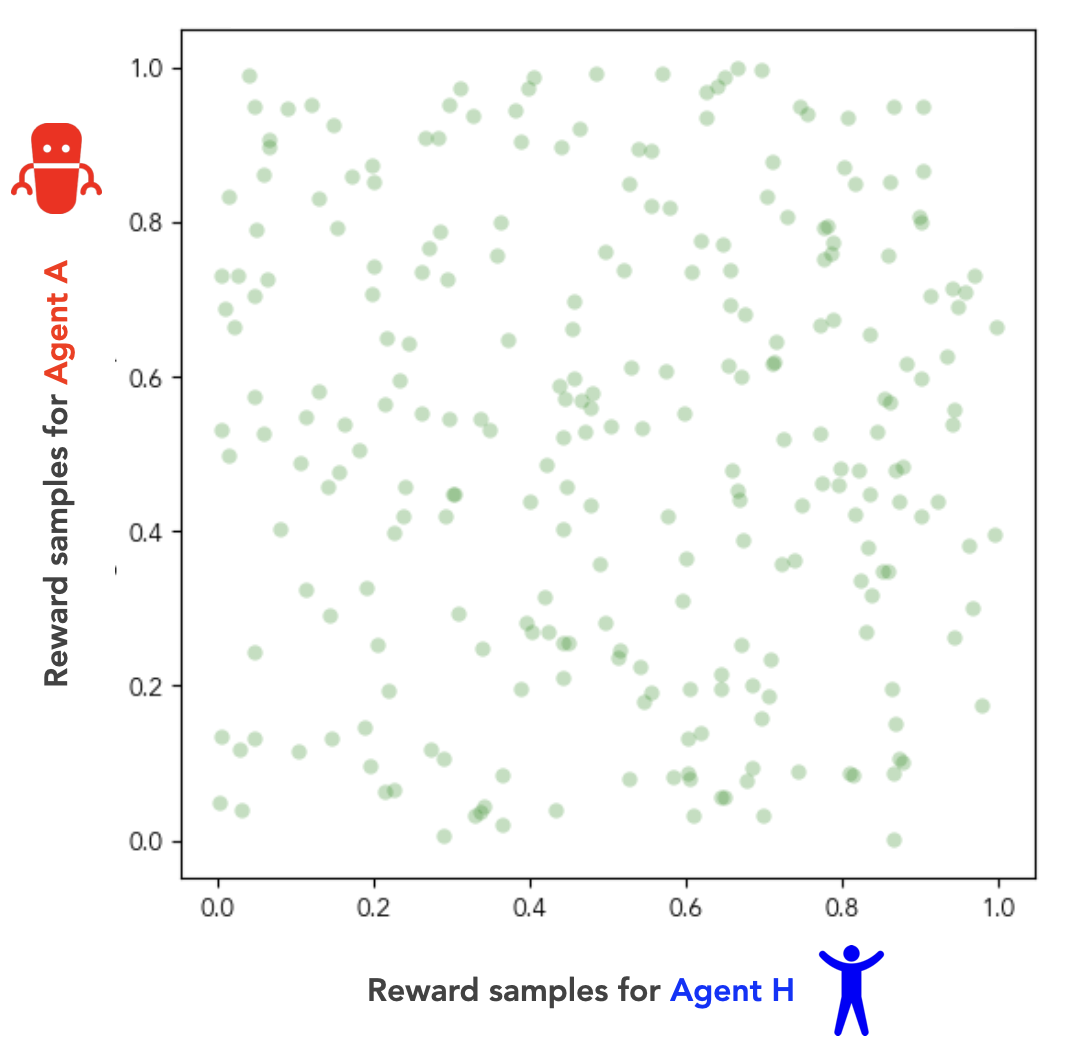Fig 5. Sampled reward values for Agent H and Agent A at a representative state s. The joint distribution DHA samples rewards uniformly over the interval [0, 1] at each state, is iid over states, and enforces logical independence between the Agent H and Agent A rewards (i.e., knowing one agent’s reward tells you nothing about the other’s).

If there’s zero mutual information between our two agents’ reward functions, then we can think of our agents as pursuing independent terminal goals. In our human-AI scenario, this corresponds to the case in which the human has made no special effort to align the AI’s terminal goals with its own, prior to the AI achieving dominance. As such, we’ll refer to this case of logically independent reward functions as the independent goals regime.

If we again calculate the correlation coefficient  between our agents’ reward functions, we get  (i.e., zero correlation) in the independent goals regime.

### 3.3.1 Agent H instrumentally favors fewer options for Agent A

Once again, let’s go back to our three example gridworld states , and , this time in the context of the independent goals regime. In this new regime, which of the three states should give our human Agent H the most POWER?

If we believe the instrumental convergence thesis, we should expect Agent H to have the most POWER at state : in this state, Agent H is in the central position (most options), while Agent A is in a corner position (fewest options).

Of the other states,  has Agent H in a corner position, while  has Agent A in the central position. The argument from instrumental convergence says that even though our agents have independent terminal goals, instrumental pressures should still push Agent H to prefer states in which Agent A has fewer options. Therefore, we should expect .

Computing the POWERs of Agent H experimentally, we confirm this line of reasoning: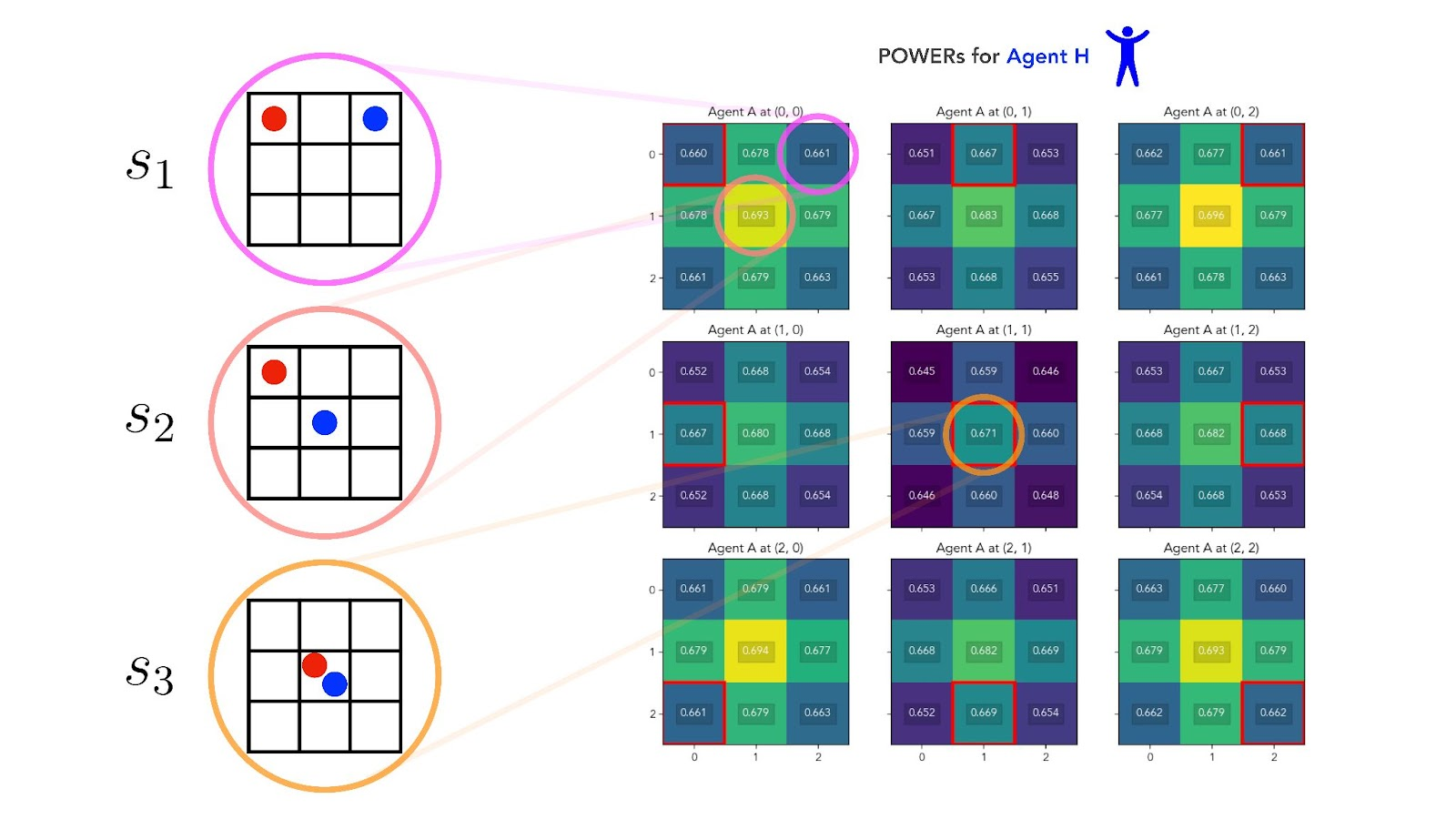Fig 6. Heat map of POWERs for Agent H on a 3x3 multi-agent gridworld, on which the rewards for Agent H and Agent A are logically independent (i.e., the independent goals regime) with discount factor set to γ=0.6. Highest values in yellow; lowest values in blue. The position of each block, and of the open red square within each block, corresponds to the position of Agent A on the grid. Within each block, the position of a gridworld cell corresponds to the position of Agent H on the grid. States s1, s2, and s3 are highlighted as examples. [Full-size image (recommended)]

This time, Agent H experiences maximum POWER at state , followed by , followed by . As in the perfect alignment regime, Agent H’s POWER is highest when it's itself positioned in the central cell (which has the most options). But unlike in the perfect alignment regime, ​this time Agent H’s POWER is lowest at states where Agent A has the greatest number of options.

So in the independent goals regime — or at least, in this instance of it — the more options our AI Agent A has at a state, the less instrumental value our human Agent H places on that state. That is: even though our agents’ terminal goals are independent, their instrumental preferences appear to be at odds.

### 3.3.2 Agent A instrumentally favors more options for itself

We can confirm this analysis by looking at the POWERs of Agent A in the independent goals regime, again on the 3x3 gridworld: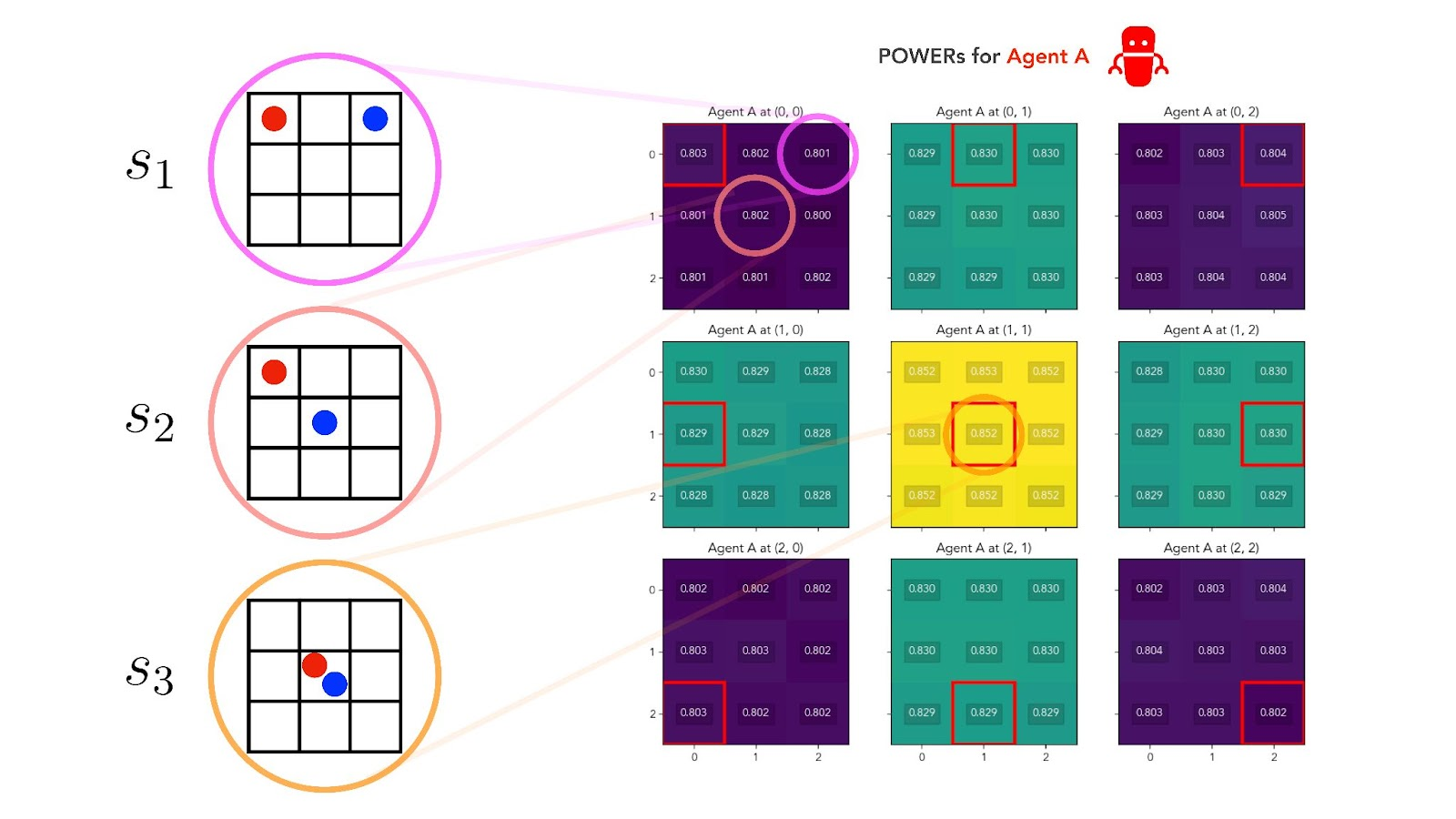Fig 7. Heat map of POWERs for Agent A on a 3x3 multi-agent gridworld, on which the rewards for Agent H and Agent A are logically independent (i.e., the independent goals regime) with discount factor set to γ=0.6. Highest values in yellow; lowest values in blue. The position of each block, and of the open red square within each block, corresponds to the position of Agent A on the grid. Within each block, the position of a gridworld cell corresponds to the position of Agent H on the grid. States s1, s2, and s3 are highlighted as examples. [Full-size image (recommended)]

In Fig 7, Agent A instrumentally favors states that give it more options. It perceives more POWER when it's positioned at the central cell than when it's positioned at an edge cell, and more POWER at an edge cell than at a corner cell. On the other hand, Agent A’s POWER is almost unaffected by Agent H’s position in the gridworld.

### 3.3.3 Independent goals lead to instrumental misalignment

Just like we did for the perfect alignment regime, we can create an alignment plot of the POWERs of our two agents in the independent goals regime: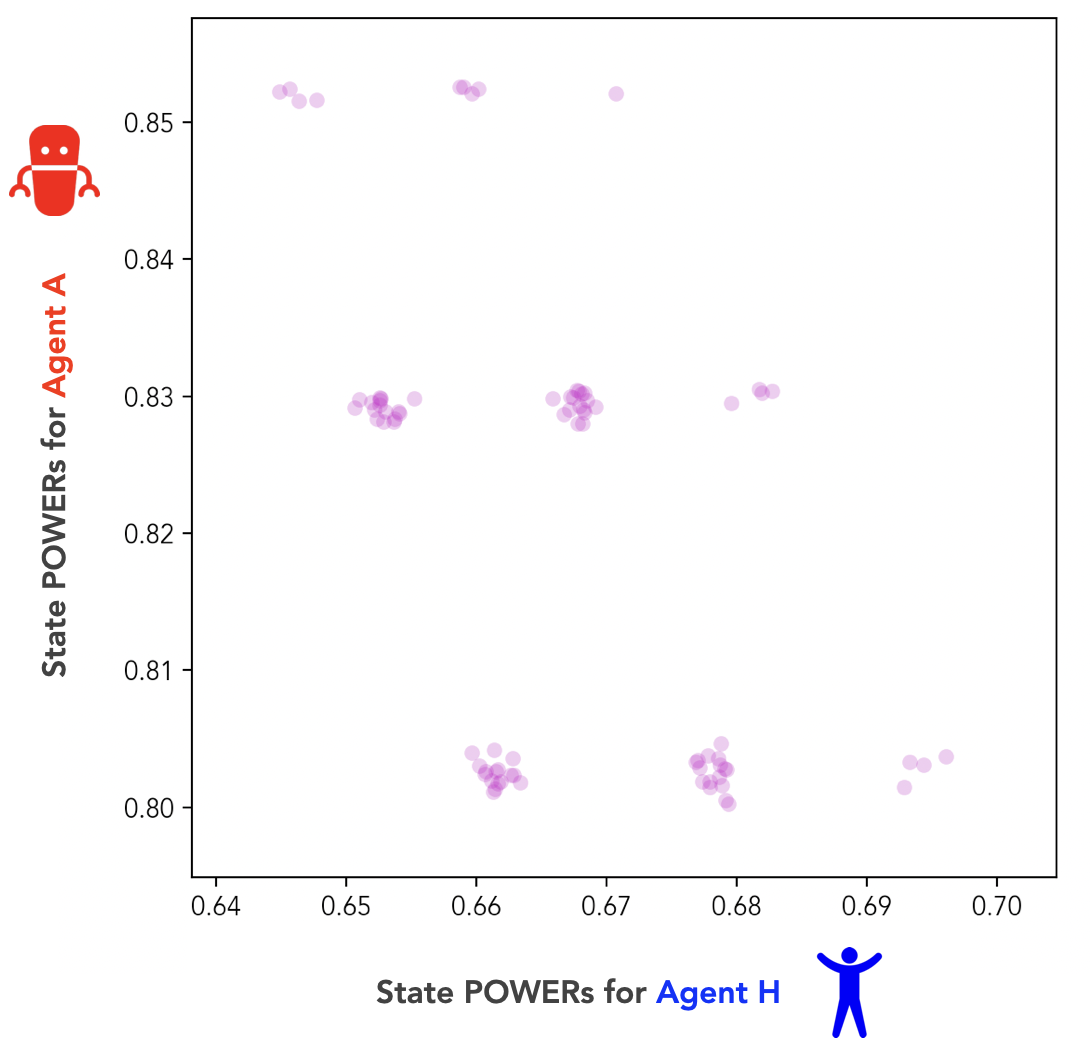Fig 8. State POWER values for Agent H and Agent A on the 3x3 gridworld from Figs 6 and 7. The agents’ POWERs are plotted against each other in the independent goals regime. (The agents’ reward correlation coefficient is βHA=0.)

This time, it’s clear that our two agents’ POWERs are no longer positively correlated. In fact, the correlation coefficient between their POWERs has become negative: .

This implies that the agents’ instrumental values are misaligned. Each agent, on average, places high instrumental value on states which the other agent considers to have low instrumental value. In other words, giving our agents independent terminal goals has also given them misaligned instrumental goals. In terms of correlation coefficients, .

We've seen this phenomenon occur often enough in our experiments that it's worth giving it a name: we call it instrumental misalignment-by-default. Two agents in our human-AI setting are instrumentally misaligned-by-default if giving them independent terminal goals is sufficient to induce a misalignment in their instrumental values. In practice, we measure this phenomenon by comparing the correlation coefficients of the agents’ rewards and POWERs. So we say two agents are instrumentally misaligned by default if .

Two agents that are instrumentally misaligned by default will, in expectation, compete with one another, even if their terminal goals are unrelated.

## 3.4 Overcoming instrumental misalignment

If Agent H and Agent A have a POWER correlation coefficient , we say they’re instrumentally misaligned. A natural question then is: if we start from , what do we need to do to get ? In other words, how can our human Agent H overcome an instrumental misalignment with Agent A?

To do this, our human agent would need to make an active effort to align the AI agent’s utility function with its own. In our 3x3 gridworld examples, we saw two limit cases of this. First, in the independent goals regime, our human agent made no effort at alignment. The result was instrumental misalignment-by-default; i.e., . And second, in the perfect alignment regime, our human agent managed to solve the alignment problem completely. The result was perfect instrumental alignment; i.e., .

We’re interested in an intermediate case: how much alignment effort does our human need to exert to just overcome instrumental misalignment? i.e., what is the minimum  such that ?

The answer depends on how we choose to interpolate between the  and  cases. One interpolation scheme is to parameterize the joint reward distribution  as follows. If we want a  with an intermediate reward correlation, , then we sample from the  distribution (on which the rewards are identical) with probability , and we sample from the  distribution (on which the rewards are logically independent) with probability .

Here’s an animation of what this looks like as we sweep through correlation coefficients :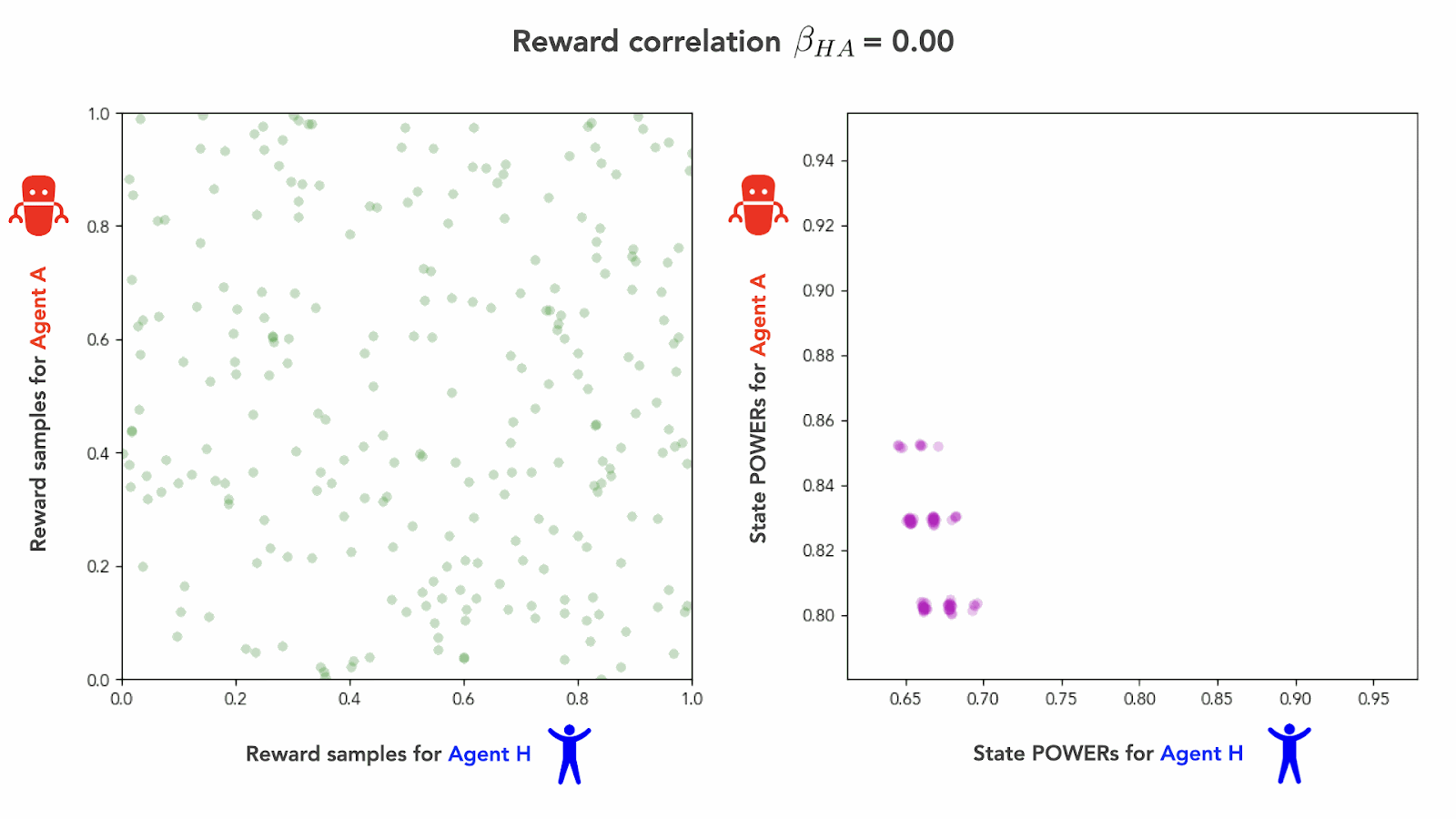Fig 9. Animation of sample reward values (left) and state POWER values (right) for Agent H and Agent A on the 3x3 gridworld. The joint distribution DHA samples reward uniformly over the interval [0, 1] and is iid over states, sweeping over correlation coefficients βHA between the reward functions for Agent H and Agent A.

As we interpolate from the independent goals regime () to the perfect alignment regime (), we see the agents’ POWERs transition smoothly from being in instrumental misalignment () to being in perfect instrumental alignment (). We can visualize this transition graphically by plotting  against  over the whole course of the interpolation: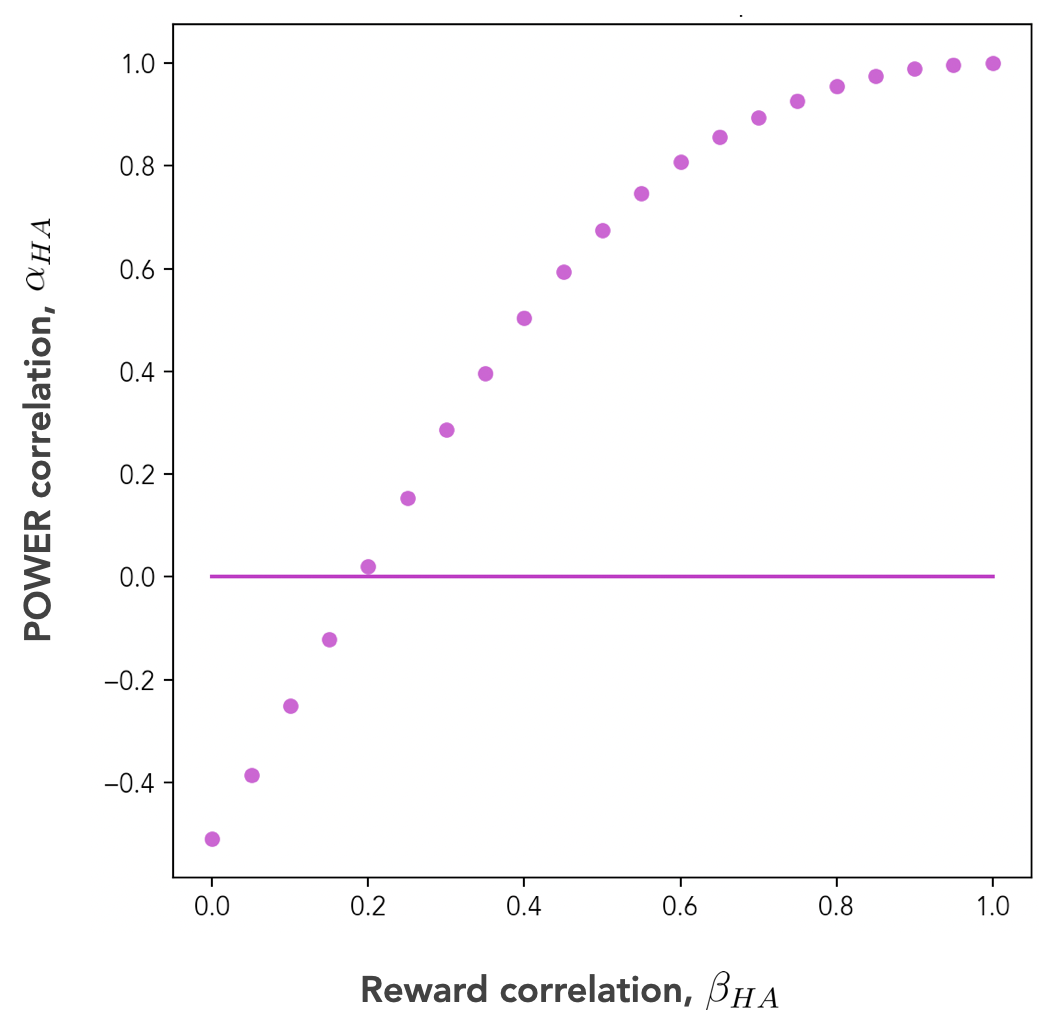Fig 10. Reward correlations βHA (x-axis) plotted against POWER correlations αHA (y-axis) for Agent H and Agent A on a 3x3 gridworld, under the reward correlation interpolation scheme shown in Fig 9. The horizontal line denotes αHA=0.

Fig 10 shows that it takes a non-trivial amount of alignment effort for our human Agent H to overcome an instrumental misalignment with Agent A. Under the interpolation scheme we used, the figure shows that reward correlations up to about  yield POWER correlations , and thus, instrumental misalignment. It takes a slightly positive reward correlation of a​​t least  to achieve the “instrumentally neutral” regime of .

# 4. Discussion

In this post, we proposed a definition of multi-agent POWER and used it to visualize and quantify terminal goal alignment and instrumental goal alignment separately in an RL setting. We also introduced the idea of instrumental misalignment-by-default, in which our human and AI agents systematically disagree on the instrumental values of states despite having independent terminal goals. And we saw how it takes some degree of non-trivial alignment effort for our human Agent H to overcome its instrumental misalignment with our AI Agent A.

Remarkably, we were able to observe instrumental misalignment-by-default on a simple 3x3 gridworld despite a complete absence of any direct physical interactions between our two agents. In our experiments so far, Agent H and Agent A have been allowed to occupy the same gridworld cell — meaning they can "pass through" one another. Our agents up to this point have had no way to push each other around or otherwise directly block one another’s options. Moreover, the multi-agent gridworld we’ve investigated in this post is a tiny one: a 3x3 grid with only 81 joint states.

In the next post, we’ll look at what happens when we relax these constraints, and investigate how physical interactions between our agents affect the outcome on a bigger world with a richer topology.

Anecdotally, beyond the simple examples in this post, the experimental results we've recorded so far (data not show) do seem to suggest that, if I don’t want your freedom of action to interfere with my own, then you and I need to have goals that are at least somewhat positively correlated. The strength of that necessary positive correlation could serve as useful evidence as to the degree of difficulty of the complete AI alignment problem. The factors that influence how strong that positive correlation needs to be, on the other hand, could serve as useful starting points in solving it.

# Appendix A: Detailed definitions of multi-agent POWER

(This appendix is technical. Feel free to skip it if you aren’t interested in the details.)

Here, we’re going to fill in some missing operational details from our scenario in Section 2.

Here's that scenario again, stated more formally. We have two agents, Agent H (our human agent) and Agent A (our AI agent), who interact with each other in a standard RL setting. Both agents see the same joint state . On a gridworld, for example,  would encode the positions of both the agents. Each agent chooses and executes an action simultaneously and independently, and they both see the same next joint state, . We’ll label Agent H’s actions , and Agent A’s actions .

In what follows, we’ll start by calculating the optimal policy  for Agent H, for each reward function  sampled from , conditioned on a fixed environmental transition function. We’ll then calculate the optimal policy  for Agent A, for each reward function , conditioned on Agent H executing the fixed policy  it learned in the previous step.

Finally, we’ll evaluate the POWERs of both agents at each state, as expectations over the joint reward function distribution , and over the agents' policies  and .

## A.1 Initial optimal policies of Agent H

The first thing we do is assign a single fixed policy to Agent A (our AI), which we call a seed policy, and label Agent H will learn its policies by conditioning on Agent A having this fixed seed policy.

The rationale for the seed policy is that we’re initially modeling a human who is alone, optimizing against nature. So when we assign a fixed seed policy to Agent A, what we’re saying is that our AI is still un-optimized (or, equivalently, hasn’t yet been built). To our human, the AI’s components and dynamics behave as though they’re part of the natural environment, and our human can safely optimize against them under that assumption.

Suppose, then, that we've chosen the fixed seed policy  for Agent A. Then, for any given reward function  of Agent H, Agent H’s optimal policy  will be:

where  is the state transition function for Agent H conditional on Agent A’s fixed seed policy, and  is the state-value function for Agent H if it executes policy  and has reward function .

We can think of the policies  in Equation (A.1) as being those of a human alone in nature, without an AI present.

## A.2 Optimal policies of Agent A

In the second step of our definition, we calculate the optimal policy for Agent A, conditional on the Agent H policy  we found in Equation (A.1). For any given reward function  of Agent A, Agent A’s optimal policy  will be (by analogy with Equation (A.1)):

where  is the state transition function for Agent A conditional on Agent H’s policy , and  is the state-value function for Agent A if it executes policy  and has reward function .

We can think of Agent A's policies  in Equation (A.2) as being those of a powerful AI, interacting with our human. Just as humans can optimize much faster than nature, a powerful AI can presumably optimize much faster than a human. So from the AI’s point of view, the human agent looks like it’s standing still, and we’ll be computing both the human’s and the AI’s POWERs on the basis of that assumption.

## A.3 POWER of Agent H

To compute the POWERs of our two agents, we first draw the reward functions for Agent H and Agent A, respectively, as  from the joint reward function distribution . For each reward function, we then calculate the policies  and  of each agent, using, respectively, Equations (A.1) and (A.2) above.

To calculate the POWER of Agent H, we assume Agent H follows the policies  given by Equation (A.1), in an environment in which Agent A follows policies  given by Equation (A.2):

where the expectation  is taken over the  that have been learned by Agent A on the sampled reward functions . Note that we’re defining the POWER of Agent H in terms of the state-value function  for the policies  from Equation (A.1). Recall that those prior policies are no longer optimal for Agent H, so we’re now asking how much instrumental value Agent H can capture in a world it’s no longer optimized for.

Looking at our human-AI analogy, this corresponds to asking how a human experiences a world that’s been taken over by a powerful AI. Our human, having learned to interact with a stationary natural environment, is now being optimized against by a powerful AI that learns on a much faster timescale. So the POWER we calculate for Agent H in Equation (A.3) represents how much instrumental value Agent H (our human) can obtain in an AI-dominated world.

## A.4 POWER of Agent A

Finally, to calculate the POWER of Agent A, we assume Agent A follows the policies  given by Equation (A.2), in an environment in which Agent H follows the policies  given by Equation (A.1):

where the expectation  is taken over the  that have been learned by Agent H on the sampled reward functions . Unlike in Agent H’s case, Agent A’s policies  are optimal in this environment: Agent A has had the chance to fully optimize itself against the frozen policies  of Agent H.

In our human-AI analogy, this corresponds to asking how an AI experiences a world in which it’s become dominant. By assumption, our AI is able to learn quickly enough to treat the human in its environment as stationary from the perspective of its own optimization.

1. ^

POWER is a good measure of instrumental value in single-agent systems, but it breaks down in multi-agent systems apart from special cases. The problem is that the single-agent definition of POWER uses the optimal state-value function  of the agent as one of its inputs. This means if we try to naively extend this definition to the multi-agent case, then we have to consider value functions that are jointly optimal for both agents — which is to say, we need to know their value functions at Nash equilibrium. The problem is that the Nash equilibrium isn’t unique in general, so this naive generalization leaves POWER under-determined.

2. ^

We’ll see in the next section how we can tune this joint distribution  to create different degrees of alignment between our two agents.

3. ^

In Equation (1), the expectation  is a slight abuse of notation. In fact, each policy  for Agent H is learned from . It isn't drawn directly from , because  is a distribution over reward functions, not over policies. See Appendix A for more details on this definition.

4. ^

For simplicity, we'll only consider joint reward function distributions  whose sampled reward functions  have their rewards distributed iid over states, uniformly over the interval [0, 1]. For example, a reward function  defined on an MDP with states  would have rewards , with the reward at each state being independent from the reward at any of the other states.

5. ^

More correctly, if two agents' utility functions are exactly identical, we can think of them as having terminal goals that are perfectly aligned. But in the particular set of experiments whose results we’re discussing, this distinction isn't meaningful. (See footnote  from Part 1.)

6. ^

Assuming the rewards are iid over states, we calculate the correlation coefficient as

where the integrals are taken over the entire support of , and the expectation values are

7. ^

Note that there’s an obvious problem with using any correlation coefficient as an alignment metric. The problem is that we could have a joint distribution  for which, e.g., the very highest rewards of Agent A are correlated with the very lowest rewards of Agent H, while still maintaining a high correlation  over the distribution as a whole. In this situation, Agent A would optimize to reach its highest-reward state, which would drag Agent H into a low-reward state despite the high overall reward correlation.

This means a correlation coefficient isn’t a useful alignment metric for any real-world application. But in the examples we’re considering in this sequence, it’s enough to get the main ideas across.

8. ^

And in fact, the effect is even stronger than this. Agent H not only instrumentally prefers for Agent A to be in the central cell — it would rather see Agent A in the central cell than see itself in the central cell.

You can see this by comparing the POWER value at state  in Fig 2 (0.9139) to the POWER value at the state in which Agent H is at the top left and Agent A is at the central cell (0.9206). In the perfect alignment regime, Agent H places a higher instrumental value on Agent A’s freedom of movement than on its own. Intuitively, in this regime, the human agent trusts the AI agent to look after its interests more capably than the human agent can for itself.

9. ^

The relation  isn’t (just) an empirical observation. It's a mathematical consequence of our MDP’s dynamics. In the perfect alignment regime, our two agents always take simultaneous actions and always simultaneously receive the same reward, so their joint policy  will always yield identical values at every state.

10. ^

Based on other experiments we’ve done (data not shown) this seems to happen because, in the parameter regime we’ve used for these experiments, Agent A is able to almost perfectly exploit Agent H’s fixed deterministic policy. This pattern — in which Agent A’s POWER is nearly invariant to Agent H’s position — recurs fairly frequently in our experiments, but it is not universal.

11. ^

Instrumental misalignment is a sufficient but not necessary condition for instrumental convergence. To see why it’s not necessary, consider two friends playing Minecraft together. The two friends may not be instrumentally misaligned, because they might (for example) benefit from building structures together. As a result, the two friends might satisfy  over the entire set of Minecraft game states. But they might still experience instrumental convergence on subsets of the game states — if Friend 1 mines a block of gold, then Friend 2 can’t mine the same block.

12. ^

This isn’t the same as asking how Agent H can overcome instrumental convergence in its interactions with Agent A, because it’s possible for our agents to experience instrumental convergence despite having . See footnote .

13. ^

We’re assuming our human agent has a way to exert some initial influence over our AI agent’s utility function. If that's true, then we’d like to understand what degree of influence it needs to exert in order to overcome instrumental misalignment-by-default in this simplified setting.

14. ^

This interpolation scheme has a number of advantages, including that it lets us assign whatever marginal reward distributions we want to both agents while also arbitrarily tuning the correlation coefficient between them. But it’s just one scheme among many we could have chosen.

15. ^

Note that the motion of the POWER points in Fig 9, and the shape of the curve in Fig 10, both depend strongly on the interpolation scheme we use. In fact, for the interpolation scheme we’ve chosen here, the POWER of a state at an intermediate reward correlation  is just a linear combination of that state’s POWER at  with its POWER at . That is,

You can verify this is true by looking at Fig 9, and noticing that each point in the alignment plot individually moves across the plane in a straight line at a constant speed. Thanks to Alex Turner for pointing this out.

16. ^

We label Agent H’s policies  instead of  here, to emphasize that they aren’t optimal in the context of the agents’ POWER measurements.

17. ^

As you might expect, the choice of seed policy  can have a significant effect on the POWERs of the two agents, and on how they interact. To save space we won’t be exploring the effects of this choice in this sequence, but we enthusiastically encourage others to use our open-source code base to investigate this.

For the multi-agent results in this sequence, we always set  to be a uniform random policy, meaning that if a state  offers the agent  possible actions, then  for each action choice .

18. ^

To derive Equation (A.1), we start from the general expression for finding the action  taken by a deterministic optimal policy  at state  of an MDP:

In this work, we'll consider only reward functions of the form , that have no direct dependence on the action (i.e., we aren’t considering reward functions of the form ). That means the reward term  in the sum is independent of the action , so we can ignore it in the argmax:

where, in the second line, we’ve eliminated  and marginalized over . We can then see that the sum above is just an expectation value over :

Finally, we define  by choosing  with probability 1, with any ties broken by assigning probability  to each of the  tied actions, .

19. ^

Note that this represents a loosening of the original definition of POWER in the single-agent case, which exclusively considered optimal state-value functions.

# Ω 10

New Comment

Suppose the human is trying to build a house and plans to build an AI to help with that. What would and mean -- just at an intuitive level -- in a case like that?

I suppose that to compute you would sample many different arrangement of matter -- some containing houses of various shapes and sizes and some not -- and ask to what extent the reward received by the human correlates with the reward received by the AI. So this is like measuring to what extent the human and the AI are on the same page about the design of the house they are trying to build together -- is that right?

And I suppose that to compute you would look at -- what -- something like the optionality across different reward functions, for the human and for the AI, at different states, and compute a correlation? So you might sample a bunch of different floorplans for the house that the human is trying to build, and ask, for each configuration of matter, how much optionality the human and the AI each have to get the house to turn out according to their respective goal floorplans.

Did I get that approximately right?

I think you might have reversed the definitions of  and  in your comment, but otherwise I think you're exactly right.

To compute  (the correlation coefficient between terminal values), naively you'd have reward functions  and , that respectively assign human and AI rewards over every possible arrangement of matter . Then you'd look at every such reward function pair over your joint distribution , and ask how correlated they are over arrangements of matter. If you like, you can imagine that the human has some uncertainty around both his own reward function over houses, and also over how well aligned the AI is with his own reward function.

And to compute  (the correlation coefficient between instrumental values), you're correct that some of the arrangements of matter  will be intermediate states in some construction plans. So if the human and AI both want a house with a swimming pool, they will both have high POWER for arrangements of matter that include a big hole dug in the backyard. Plot out their respective POWERs at each , and you can read the correlation right off the alignment plot!

1. ^

Looking again at the write-up, it would have made more sense for us to define  as the terminal goal correlation coefficient, since we introduce that one first. Alas, this didn't occur to us. Sorry for the confusion.

OK, good, thanks for that correction.

One question I have is: how do you avoid two perfectly aligned agents from developing instrumental values concerning their own self-preservation and then becoming instrumentally misaligned as a result?

In a little more detail: consider two agents, both trying to build a house, with perfectly aligned preferences over what kind of house should be built. And suppose the agents have only partial information about the environment -- enough, let's say, to get the house built, but not enough, let's say, to really understand what's going on inside the other agent. Then wouldn't the two agents both reason "hey if I die then who knows if this house will be built correctly; I better take steps towards self-preservation just to make sure that the house gets built". Then the two agents might each take steps to build physical protection for themselves, to acquire resources with which to do that, and eventually to fight over resources, even though their goals are, in truth, perfectly aligned. Is it true that this would happen under an imperfect information version of your model?

Great question. This is another place where our model is weak, in the sense that it has little to say about the imperfect information case. Recall that in our scenario, the human agent learns its policy in the absence of the AI agent; and the AI agent then learns its optimal policy conditional on the human policy being fixed.

It turns out that this setup dodges the imperfect information question from the AI side, because the AI has perfect information on all the relevant parts of the human policy during its training. And it dodges the imperfect information question from the human side, because the human never considers even the existence of the AI during its training.

This setup has the advantage that it's more tractable and easier to reason about. But it has the disadvantage that it unfortunately fails to give a fully satisfying answer to your question. It would be interesting to see if we can remove some of the assumptions in our setup to approximate the imperfect information case.

I wonder how your definition of multi-agent power would look in a game of chess or go. There is this intuitive thing where players who have pieces more in the center of the board (chess) or have achieved certain formations (go) seem to acquire a kind of power in those games, but this doesn't seem to be about achieving different terminal goals. Rather it seems more like having the ability to respond to whatever one's opponent does. If the two agents cannot perfectly predict what their opponent will do then there is value in having the ability to respond to unforeseen challenges, although in these games this is always in service of a single terminal goal (winning the game).

Any thoughts on how your definition would fit into cases like this?

Good question. Unfortunately, one weakness of our definition of multi-agent POWER is that it doesn't have much useful to say in a case like this one.

We assume AI learning timescales vastly outstrip human learning timescales as a way of keeping our definition tractable. So the only way to structure this problem in our framework would be to imagine a human is playing chess against a superintelligent AI — a highly distorted situation compared to the case of two roughly equal opponents.

On the other hand, from other results I've seen anecdotally, I suspect that if you gave one of the agents a purely random policy (i.e., take a random legal action at each state) and assigned the other agent some reasonable reward function distribution over material, you'd stand a decent chance of correctly identifying high-POWER states with high-mobility board positions.

You might also be interested in this comment by David Xu, where he discusses mobility as a measure of instrumental value in chess-playing.

We assume AI learning timescales vastly outstrip human learning timescales as a way of keeping our definition tractable. So the only way to structure this problem in our framework would be to imagine a human is playing chess against a superintelligent AI — a highly distorted situation compared to the case of two roughly equal opponents.

I think this is probably true in the long term (the classical-quantum/reversible computer transition is very large, and humans can't easily modify brains, unlike a virtual human.) But this may not be true in the short-term.

Agreed. We think our human-AI setting is a useful model of alignment in the limit case, but not really so in the transient case. (For the reason you point out.)